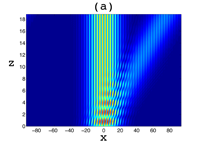April 2011
Mon Tue Wed Thu Fri Sat Sun
« Mar   May »
123
45678910
11121314151617
18192021222324
252627282930

## PT-Symmetric Sinusoidal Optical Lattices at the Symmetry-Breaking Threshold

Eva-Maria Graefe, H. F. JonesThe PT-symmetric potential $$V_0[\cos(2\pi x/a)+i\lambda\sin(2\pi x/a)]$$ has a completely real spectrum for $$\lambda\le 1$$, and begins to develop complex eigenvalues for $$\lambda>1$$. At the symmetry-breaking threshold $$\lambda=1$$ some of the eigenvectors become degenerate, giving rise to a Jordan-block structure for each degenerate eigenvector. In general this is expected to result in a secular growth in the amplitude of the wave. However, it has been shown in a recent paper by Longhi, by numerical simulation and by the use of perturbation theory, that for a broad initial wave packet this growth is suppressed, and instead a saturation leading to a constant maximum amplitude is observed. We revisit this problem by explicitly constructing the Bloch wave-functions and the associated Jordan functions and using the method of stationary states to find the dependence on the longitudinal distance $$z$$ for a variety of different initial wave packets. This allows us to show in detail how the saturation of the linear growth arises from the close connection between the contributions of the Jordan functions and those of the neighbouring Bloch waves.

http://arxiv.org/abs/1104.2838
Optics (physics.optics); Quantum Physics (quant-ph)